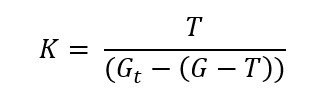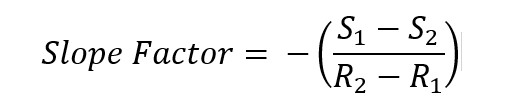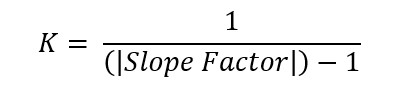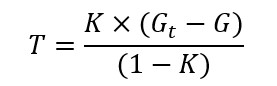# Thickness Measurement of Dielectric Materials

### — Description —

#### Introduction

Many applications, both in manufacturing and research, require precise material thickness measurements. This becomes especially difficult if the material is moving, is non conductive and/or translucent to clear.

The specifics of your application may make accurate measurements difficult; many circumstances prohibit contact measurement, and make laser measurements difficult or impossible, for example with thin clear materials such as glass, sapphire, plastic .

In cases such as these, capacitance measurement provides a highly accurate and cost-effective solution. While other measurement systems such as eddy current probes are incompatible with dielectric materials, the MTI Accumeasure (paired with MTI dielectric probes) provides touch-free and high-resolution thickness measurements with some simple calculations.

For some applications, optical and laser systems can provide highly accurate thickness measurements. These systems are not universally applicable as vibration, surface defects, and variations in material reflectiveness can effect accuracy.

The Accumeasure capacitance measurement system, however, provides accurate measurements despite these conditions. Additionally, Accumeasure products can easily integrate into a variety of existing laboratory or production systems, offer multi-unit synchronization, and have a proven history of accuracy, stability, and durability.

#### Dielectric Challenges

Due to the physics of capacitance measurement, systems are easily configured for measuring both conductive and non-conductive materials. Almost all non-conductive material are dielectric materials, by their nature, require additional calibrations and calculations.¬† Using the simple methods bellow, MTI Accumeasure systems can provide the same accuracy for dielectric uses. These mathematical methods serve to demonstrate the calculations necessary for capacitance sensors if only a voltage output is available.

MTI‚Äôs Digital Accumeasure Series can directly measure non-conductive material thickness with the free Digital Accumeasure Measurements Program ¬†. No calculations are necessary; however , if you are using MTI‚Äôs legacy Amplifiers such as the Accumeasure 9000, MicroCap or AS-563 analog capacitive amplifiers then you will need to convert the amplifiers voltage output to thickness with a few simple calculations as given below.

### — Technical Notes —

#### Understanding the Physics

Despite being electrical insulators, dielectric materials have the capability to store electric charge. In other words, electrons will not flow through the material but do shift, giving the material a slight electric polarization.¬† The effect of this polarization depends partly on the unique properties of each material ‚Äì specified by the material‚Äôs Dielectric Constant. The value of the dielectric constant directly affects capacitance measurements; by determining the value for your material, accurate capacitance measurements can be obtained.

For the more interested the dielectric constant is related to the thickness by the Capacitance formula:

C= ŒµA/S

Where:

C = capacitance

Œµ = dielectric constant of the insulator

A = area of the probe sensing element

S = gap from probe face to the ground plateFigure 1: When we calculate d the thickness of the insulator in Figure 1 we need to ignore the gap S-d which is air. The formulas given below do just that.

#### Determining the Dielectric Constant ‚Äì Single Sample Method

If the dielectric constant ( K ) ¬†¬†is unknown, it can be easily determined using a representative material sample of known thickness ( T ).¬† In this case a one-time contact measurement of sufficient accuracy can be used (ensure a resolution of at least 2 microns).

Arrange the dielectric probe to provide a gap at least twice as wide as the sample material‚Äôs thickness. Using the Accumeasure Capacitance Sensor, take one reading without the sample material, ( G ) and one reading with the sample material inserted in the probe gap ( Gt ).

The material dielectric constant ¬†can be determined by the equation:#### Determining the Dielectric Constant ‚Äì Slope Factor (Two Sample) Method

Given two dielectric samples (same dielectric constant) of known thickness1 (either provided by the sample manufacturer or measured with micrometers), a new amplifier slope-factor (sensitivity factor) can be determined by the equation:Where S1 and S2 are the known thickness of the prospective samples, and R1 and R2 are the corresponding Accumeasure readings. The dielectric constant ( K ) is then:#### Thickness Measurement ‚Äì Dielectric Constant and Probe Gap are Known

Once the Dielectric Constant ( K ) is known (either as a known property or by using the methodology above), the thickness of other samples of the material can easily be calculated using the analog Accumeasure.

Again ensure the probe gap is at least twice the material thickness, and take baseline (without material inserted) Accumeasure reading ( G ). By taking readings with the material inserted ( Gt ), the material thickness ( T ) can be calculated by:Notes 1:¬† The material thickness should be known to an accuracy of 1 um.

Are you looking for process control metrology products?¬† MTI‚Äôs systems can measure thickness, total thickness variation, bow and warp as part of in-process monitoring or as a quality station in production.